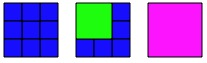#### You may also like### Pebbles

Place four pebbles on the sand in the form of a square. Keep adding as few pebbles as necessary to double the area. How many extra pebbles are added each time?### It Figures

Suppose we allow ourselves to use three numbers less than 10 and multiply them together. How many different products can you find? How do you know you've got them all?### Bracelets

Investigate the different shaped bracelets you could make from 18 different spherical beads. How do they compare if you use 24 beads?

# Tiling

##### Age 7 to 11Challenge Level

Let's imagine we are tiling a floor or patio area.
Suppose the area is square and is 3 by 3.

Tiles come in three sizes: 1 by 1, 2 by 2 and 3 by 3.
You are allowed to use any arrangement of these tiles to cover the space completely.
However, none of the tiles can be cut.

For this challenge, we are interested in the total number of tiles you use for any arrangement.
For example, for the 3 by 3 square you could do it by using:nine 1 by 1 tiles,

OR

one 2 by 2 and five 1 by 1 tiles,

OR

one 3 by 3 tile.

Therefore the smallest number of tiles is one and the largest is nine and the only other value you can get between one and nine is six (using one 2 by 2, and five 1 by 1).

Now imagine you also have tiles which are 4 by 4 in size.
What total numbers of tiles can you use for a square patio that is 4 by 4?
If you could have tiles which are 5 by 5 as well, what total numbers of tiles can you use for a square patio that is 5 by 5?

The final part of this activity is to examine carefully the answers you get for each of the three sizes of floors - 3 by 3, 4 by 4 and 5 by 5.

Now talk about and record what you notice.
Make some statements about what you therefore think will happen if someone takes the long time needed to explore a 6 by 6 and 7 by 7 square.